Next: Two-body problem Up: Newtonian mechanics Previous: Motion in one-dimensional potential

# Simple harmonic motion

Consider the motion of a point particle of mass, moving in one dimension, that is slightly displaced from a stable equilibrium point located at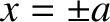. Suppose that the particle is moving in the conservative force field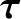. According to the preceding analysis, in order forto correspond to a stable equilibrium point, we require both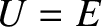(2.56)

and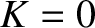(2.57)

Our particle obeys Newton's second law of motion,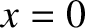(2.58)

Let us assume that the particle always stays fairly close to its equilibrium point. In this case, to a good approximation, we can representvia a truncated Taylor expansion about this point. In other words,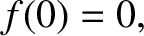(2.59)

However, according to Equations (2.56) and (2.57), the preceding expression can be written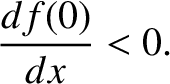(2.60)

where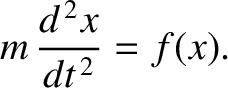. Hence, we conclude that our particle satisfies the following approximate equation of motion,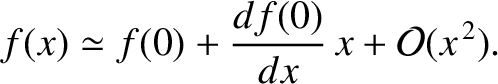(2.61)

provided that it does not stray too far from its equilibrium point; in other words, provided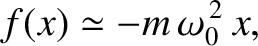does not become too large.

Equation (2.61) is called the simple harmonic equation; it governs the motion of all one-dimensional conservative systems that are slightly perturbed from some stable equilibrium state. The solution of Equation (2.61) is well known: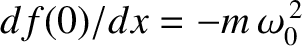(2.62)

The pattern of motion described by this expression, which is called simple harmonic motion, is periodic in time, with repetition period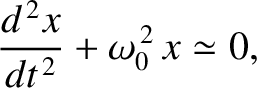, and oscillates between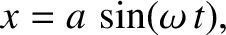. Here,is called the amplitude of the motion. The parameter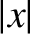, known as the phase angle, simply shifts the pattern of motion backward and forward in time. Figure 2.6 shows some examples of simple harmonic motion. Here,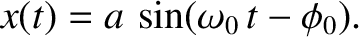,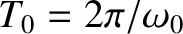, and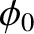correspond to the solid, short-dashed, and long-dashed curves, respectively.

Note that the frequency,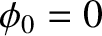--and, hence, the period,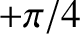--of simple harmonic motion is determined by the parameters appearing in the simple harmonic equation, Equation (2.61). However, the amplitude,, and the phase angle,, are the two integration constants of this second-order ordinary differential equation, and are thus determined by the initial conditions; that is, the particle's initial displacement and velocity.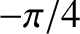From Equations (2.45) and (2.60), the potential energy of our particle at positionis approximately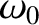(2.63)

Hence, the total energy is written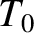(2.64)

giving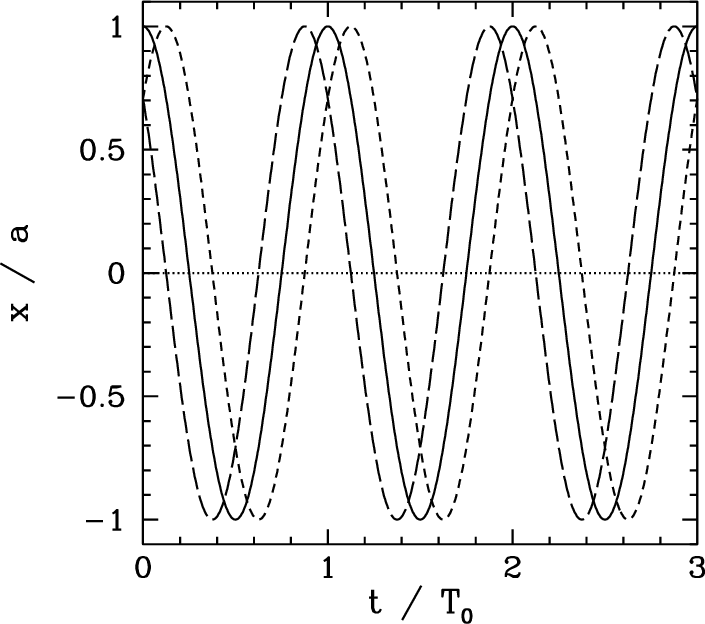(2.65)

where use has been made of Equation (2.62), and the trigonometric identity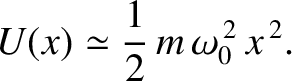. Note that the total energy is constant in time, as is to be expected for a conservative system, and is proportional to the amplitude squared of the motion.

Consider the motion of a point particle of massthat is slightly displaced from a unstable equilibrium point at. The fact that the equilibrium is unstable implies that(2.66)

and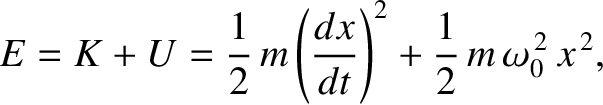(2.67)

As long asremains small, our particle's equation of motion takes the approximate form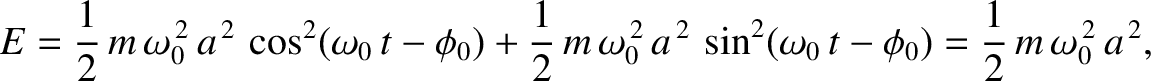(2.68)

which reduces to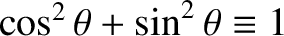(2.69)

where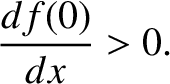. The most general solution to the preceding equation is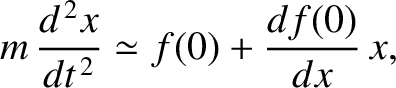(2.70)

where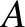and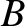are arbitrary constants. Thus, unless the initial conditions are such thatis exactly zero, the particle's displacement from the unstable equilibrium point grows exponentially in time.Next: Two-body problem Up: Newtonian mechanics Previous: Motion in one-dimensional potential
Richard Fitzpatrick 2016-03-31